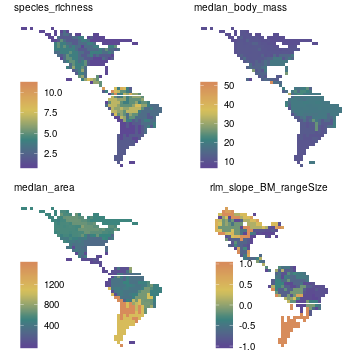## Appendix S3

Supporting information in Valcu, M., Dale, J., and Kempenaers, B. (2012). rangeMapper: a platform for the study of macroecology of life-history traits. Global Ecology and Biogeography 21, 945-951.

The example shown here is run on the wrens dataset which is part of the package. The wrens dataset has 84 species while the case study presented in the paper was run on 8434 bird species. Therefore both the settings and the results shown below are not identical with the results presented in Valcu et al 2012.

### Project Set Up

For a step-by-step project set-up see Case study 1.

``````require(rangeMapper)
"extdata", "wrens", "vector_combined"), "wrens", verbose = FALSE)
data(wrens)
d = subset(wrens, select = c('sci_name', 'body_mass') )
con = ramp("wrens.sqlite", gridSize = 2.5, spdf = breding_ranges,
biotab = d, ID = "sci_name",metadata = rangeTraits()['Area'],
FUN = "median", overwrite = TRUE)
``````
``````## New session 2019-10-25 17:52:26
``````
``````## PROJECT: wrens.sqlite
## DIRECTORY: /tmp/Rtmpe4D7pf
``````
``````## Grid size set to 2.5 map units.
``````
``````## Canvas uploaded.
``````
``````## Writing overlay output to project...
``````
``````## 84 out of 84 ranges updated; Elapsed time: 0 mins
``````
``````## Extracting metadata...
``````
``````## Table biotab saved as a  BIO_ table
``````
``````## +______________+
``````
``````## class            rangeMap
## Project_location /tmp/Rtmpe4D7pf/wrens.sqlite
## Proj4            +proj=longlat +ellps=GRS80 +towgs84=0,0,0,0,0,0,0 +no_defs
## CellSize         2.5
## Extent           xmin=-164.8716,xmax=-34.60225,ymin=-55.72056,ymax=61.5632
## BIO_tables       biotab
## MAP_tables       species_richness;median_body_mass
``````
``````## +______________+
``````

Convert `metadata_ranges` table to a life-history `BIO_` table.

``````metadata2bio(con)
``````
``````## Table metadata_ranges saved as a  BIO_ table
``````

Merge the newly converted `metadata_ranges` to the other life-history table(s) previously saved.

``````bio.merge(con, tableName = 'all_life_history')
``````
``````## Warning in result_fetch(res@ptr, n = n): SQL statements must be issued with
## dbExecute() or dbSendStatement() instead of dbGetQuery() or dbSendQuery().

## Warning in result_fetch(res@ptr, n = n): SQL statements must be issued with
## dbExecute() or dbSendStatement() instead of dbGetQuery() or dbSendQuery().
``````
``````## data frame with 0 columns and 0 rows
``````

### Define New Functions

The newly defined function should take `formula` & `data` as arguments and should return a numeric vector of length 1. We define a simple wrapper around the `rlm` function in `MASS` package to extract a robust regression slope for each assembladge (i.e. canvas cell).

Note that:

• `...` in `rangeMap.save` allows for further arguments to be passed to `rlm()`

• `try()` allows the computation to move on to the next canvas cell without stopping the entire process.

• the function will return NA if `rlm` does not return a coefficient.

``````rlm_slope = function (formula, data,...) {
x = try(as.numeric(
MASS::rlm(formula, data,...)\$coefficients), silent = TRUE)
if(inherits(x, "try-error")) x = NA
return(x)
}
``````

Map the Area ~ Body Mass slope using the user-defined function

``````rangeMap.save(con, FUN = rlm_slope, biotab = "all_life_history",
biotrait  = "body_mass_biotab",
tableName = "rlm_slope_BM_rangeSize",
maxit = 20)
``````
``````##  TRUE
``````

Additionally map the breeding range area

``````rangeMap.save(con, FUN = 'median', biotab = "all_life_history",
``````##  TRUE
``````m= rangeMap.fetch(con, spatial = FALSE,